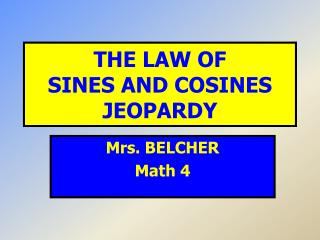DownloadDownload PresentationTHE LAW OF SINES AND COSINES JEOPARDY

# THE LAW OF SINES AND COSINES JEOPARDY

Télécharger la présentation## THE LAW OF SINES AND COSINES JEOPARDY

- - - - - - - - - - - - - - - - - - - - - - - - - - - E N D - - - - - - - - - - - - - - - - - - - - - - - - - - -

1. ### THE LAW OF SINES AND COSINES JEOPARDY

Mrs. BELCHER Math 4
2. Directions Each person should work out every problem on their paper Rotate through your group who works the problem on the white board and displays the answer to earn points Don’t hold up your white board until I ask for them Every team that gets the correct answer earns points Each team needs to keep their own score
3. JEOPARDY
4. SINE OF THE TIMES 100 If A = 63°, B = 19°, and a = 2.4, find b to the nearest tenth using the Law of Sines. 0.9 C. 6.6 0.7 D. 3.6
5. SINE OF THE TIMES 200 If B = 14°, C = 45°, and b = 5.2, find c to the nearest tenth using the Law of Sines. 1.8 C. 15.2 6.1 D. 0.9
6. SINE OF THE TIMES 300 If a = 12, B = 27°, and b = 15, find A to the nearest degree using the Law of Sines. 132° C. 35° 21° D. 118°
7. SINE OF THE TIMES 400 If A = 50°, b = 10, and a = 14, find C to the nearest degree using the Law of Sines. 57° C. 33° 73° D. 97°
8. IT’S OK… THEY’RE COSINES 100 If a = 21.5, b = 13, and C = 39°, find c to the nearest tenth using the Law of Cosines. 7.5 C. 21.9 14.1 D. 4.4
9. IT’S OK… THEY’RE COSINES 200 If A = 52°, b = 54 and c = 49, find a to the nearest tenth using the Law of Cosines. 3.9 C. 45.3 56.2 D. 78.6
10. IT’S OK… THEY’RE COSINES 300 If a = 3, b = 7 and c = 5, find B to the nearest degree using the Law of Cosines. 120° C. 22° 38° D. 90°
11. IT’S OK… THEY’RE COSINES 400 If a = 5, b = 6, and c = 8, find A to the nearest degree using the Law of Cosines. 141° C. 39° 124° D. 20°
12. HOW MANY SOLUTIONS 100 Determine the number of possible solutions, given a = 33, b = 50 and A = 132° None One Two
13. HOW MANY SOLUTIONS 200 Determine the number of possible solutions, given b = 150, a = 125 and A = 25° None One Two
14. HOW MANY SOLUTIONS 300 Determine the number of possible solutions, given A = 38°, B = 63°, and c = 15 None One Two
15. HOW MANY SOLUTIONS 400 Determine the number of possible solutions, given b = 15, c = 13, and C = 50° None One Two
16. KEEP ON A SOLVIN’ 100 In order to solve the triangle given b = 40, c = 45, and A = 51°, which equation should you use first to solve. A. a² = b² + c² - 2bc·cos A b² = a² + c² - 2ac·cos B c² = a² + b² - 2ab·cos C D. Law of Sines
17. KEEP ON A SOLVIN’ 200 If B = 41°, C = 52°, and c = 27, find a to the nearest tenth. 22.5 C. 37.6 34.2 D. 23.9
18. KEEP ON A SOLVIN’ 300 If A = 52°, b = 12, and c = 16, find B to the nearest degree. 80° C. 85° 43° D. 48°
19. KEEP ON A SOLVIN’ 400 If A = 53°, b = 40, and c = 49, find C to the nearest degree. 15° C. 75° 52° D. 83°
20. CORRECT!!!! GOOD JOB!!! GO BACK TO THE BOARD AND CHOOSE ANOTHER QUESTION!!!
21. INCORRECT!!!! SORRY! GO BACK TO THE QUESTION AND TRY AGAIN!
22. CORRECT!!!! GOOD JOB!!! GO BACK TO THE BOARD AND CHOOSE ANOTHER QUESTION!!!
23. INCORRECT!!!! SORRY! GO BACK TO THE QUESTION AND TRY AGAIN!